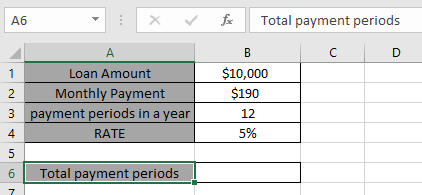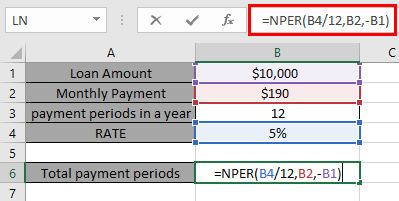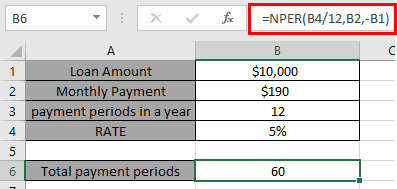# How to use the NPER Function in Excel

In this article, we will learn how to use the NPER function to calculate periods on payments in Excel.
Excel let’s a person find total monthly installment periods  on a loan amount using the function having principle amount or loan amount, interest rate per period, installment amount & number of periods in a year.
The function calculates the payment periods for a loan based on constant payments and a constant interest rate.
Syntax:

=NPER (rate, pmt, pv, [fv], [type])

Syntax for loan calculation formula:-
rate – The interest rate per period.
pmt – installment, amount paid on each period.
pv – The present value, the total amount that a series of future payments is worth now.
[fv] - [optional] pending amount after the loan. If omitted, default is zero.
[type] – [optional] The timing of the payment, either at the beginning or end of the period. Numbers 0 or 1 represent the payment date. The number 0 represents payment at the end of the period, and the number 1 represents
payment at the beginning of the period. The default (empty argument) is 0. The calculation considers payment is at the end of the period.

All of the above might be confusing for some people, so let’s gear up & start learning its usage in excel with the example.

Consider a scenario, John has taken a loan of \$10,000 from a bank at 5% per year interest, paying \$190 every month. He needs to find out his total number of payments on the loan.

So he added the following details in Excel.Here the John is paying every month. So there would be 12 payments a year.
Use the formula in B6 cell

=NPER(B2/12,C2,A2)Here all the arguments to the function is given as cell reference.
You must be wondering why Interest rate is divided by 12 as there are 12 periods of payment in a year &John has to pay \$190 per month till 60 months. You must be wondering why this amount in the function taken as negative. The loan amount is positive value which is credited to John. He has to pay that amount which is to be debited.
Hope you understood how to calculate the number of  installment periods on a loan using NPER function. Explore more articles here on calculating financial functions here. Please state your queries in the comment box below.

Related Articles

How to Use RATE Function in Excel

How to Use IRR function in Excel

Excel IPMT Function

Excel CUMIPMT Function

Popular Articles

Edit a dropdown list

If with conditional formatting

If with wildcards

Vlookup by date

Terms and Conditions of use

The applications/code on this site are distributed as is and without warranties or liability. In no event shall the owner of the copyrights, or the authors of the applications/code be liable for any loss of profit, any problems or any damage resulting from the use or evaluation of the applications/code.